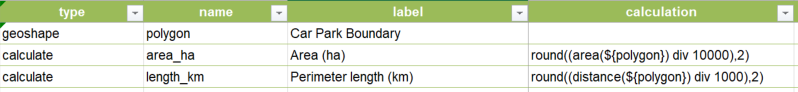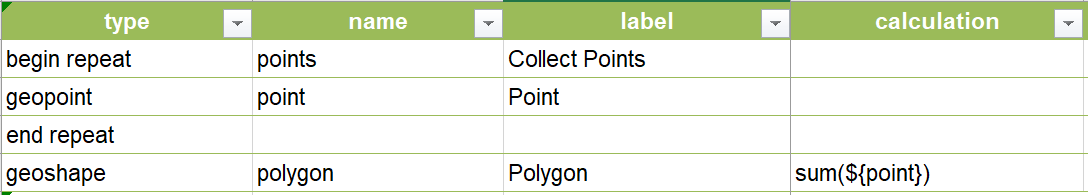# geoshape type

1467
8
03-20-2019 01:54 PMbyMVP Frequent Contributor

Can the area value of a geoshape type be used in a calculation for another field?

We need to determine the area of any polygon created and set that value on another field.

Survey123 Connect v3.3.51

1 Solution

Accepted SolutionsbyEsri Contributor

Hi James,

Sure can! You can use the area() function in a calculation field for another question. This example shows the area (in square metres) and perimeter (in metres) of a polygon being converted to hectares and kilometres and rounded to 2 decimal places.Brett

8 RepliesbyEsri Contributor

Hi James,

Sure can! You can use the area() function in a calculation field for another question. This example shows the area (in square metres) and perimeter (in metres) of a polygon being converted to hectares and kilometres and rounded to 2 decimal places.BrettbyMVP Frequent Contributor

Score!  Thanks so much for the example!by Anonymous User
Not applicable

Hello Brett StokesI have multiple GPS points. Can I do manually type with Multiple GPS Points to create a polygon in geoshape?  If we can create a polygon let me know how to create itbyEsri Contributor

Hello Thant Zaw Oo,

You can achieve this by putting your geopoint questions within a repeat. Then just use the sum() function in your geoshape question in the calculate column to build the polygon from the points in your repeat. I suggest joining the Early Adopter Community (if you haven't already) where you can find more detailed explanations on this beta functionality. Here is an example of setting up your XLSForm:Hope this helps,

Brettby Anonymous User
Not applicable

Thanks Brett, I could manage it and get polygon well. May I know same questions that I would like to know Sq Meter from this polygon for calculation If you don't mind can you please share me. Thanks.New Contributor II

Hi Thant,

did u managed to calculate the SQM of the polygon so far

ThanksbyEsri Esteemed Contributor

The area() function provides the area in square meters; no conversion is necessary.  Please refer to Geotrace and geoshape—Survey123 for ArcGIS | DocumentationNew Contributor III

Hi James,

I have a geoshape within a repeat, I can easily return the area of the geoshape in square feet within another question in my survey with the area function if  I add a single geoshape polygon using this calculation:

if(\${dstrb_area},area(\${dstrb_area})*10.7639, 0)

If I add two geoshapes in the repeat the calculation completes for the second of the two rather than for both.

How would I go about calculating the sum of two or more polygons collected in the geoshape repeat?

Thanks,